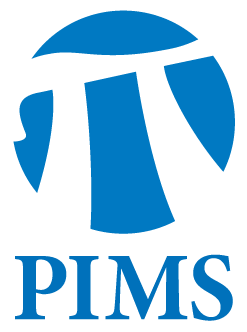PIMS/CSC Seminar: Deniz Sezer (Calgary)

• Date: 09/23/2011
• Time: 14:30
Lecturer(s):
Deniz Sezer (Calgary)
Location:

Simon Fraser University

Topic:

The Martin boundary theory for the nonlinear p.d.e.1/2 \Delta u=2 u^2: A probabilistic approach

Description:

Abstract:

The classical Martin boundary is concerned with harmonic functions: the non-negative solutions of Laplace's equation 1/2\Delta u=0 in a given domain D of R^n.  Harmonic functions are extensively studied and well understood. A culminating result is the integral representation of harmonic functions in terms of the so-called Martin kernel. Can one develop a similar theory for the nonlinear p.d.e.  1/2 \Delta u=2 u^2 as well? A key feature of the Laplace's equation is that it renders itself to probabilistic analysis, and as a result many important results on this p.d.e can be re-formulated probabilistically in terms of Brownian motion.  This not only brings new insights into the theory, but also inspires similar ideas to be used for other p.d.e.'s.  A striking example of this is the formulation of the solutions of the non-linear equation 1/2 \Delta u=2 u^2 in terms of Super-Brownian motion (SBM). Indeed most of the recent progress on understanding the solutions of this p.d.e has been made using probabilistic methods. Martin boundary theory for Laplace's equation has an important probabilistic interpretation; it tells us about the exit behavior of Brownian motion from a domain. A new research program, initiated by E.B. Dynkin, aims to build an analogous theory for SBM by employing the ideas used in the probabilistic construction of Martin boundary for Brownian motion.  In this talk I will discuss some of these key ideas and recent results that we have obtained as a part of this research program. 008, he held a Killam Research Fellowship awarded by the Canada Council for the Arts. His research interests are in differential geometry, partial differential equations and mathematical physics.

Other Information:

Taking place in room K9509.

For further information, please see the event page at: http://www.math.sfu.ca/events.